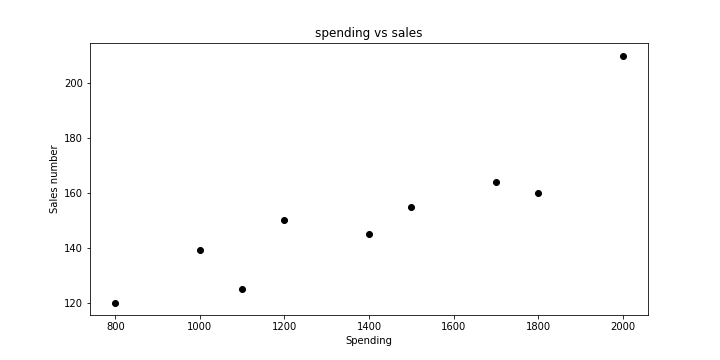# Linear Regression Model Building

## Linear Regression Model Using Python

In this blog, we will build a simple linear regression model step by step using Python and Scikit-learn library.

As the below example builds a model on a sample dataset, this does not contain any junk, null and other outliers. Subsequently, we will use more complex datasets to illustrate the process of cleaning and model building. Follow the below steps to create a linear regression model in python.

```
import pandas as pd
```

Output:

``````   ad_spending 	Sales
0 	1000 	139
1 	1500 	155
2 	1800 	160
3 	2000 	210
4 	800 	120``````
##### Step-2: Check the column details.
```
sales.info()
```

Output:

``````RangeIndex: 9 entries, 0 to 8
Data columns (total 2 columns):
Sales          9 non-null int64
dtypes: int64(2)
memory usage: 224.0 bytes``````
##### Step-3: Verify the shape of the dataframe.
```
sales.shape
```

Output:

``(9, 2)``
##### Step-4: Verify the statistical properties of the dataframe.
`sales.describe()`

Output:

``````       ad_spending 	Sales
count 	9.000000 	9.000000
mean 	1388.888889 	152.000000
std 	398.260105 	26.353368
min 	800.000000 	120.000000
25% 	1100.000000 	139.000000
50% 	1400.000000 	150.000000
75% 	1700.000000 	160.000000
max 	2000.000000 	210.000000 ``````
##### Step-5: Visualise data in the notebook.
``` import matplotlib.pyplot as plt
%matplotlib inline

fig=plt.figure(figsize=(10, 5))
plt.xlabel('Spending')
plt.ylabel('Sales number')
plt.title('spending vs sales')```

Output:##### Step-6: Split data into dependent(X) and independent(y) variable.
```
# Putting feature variable to X
X = sales.drop(['Sales'],axis=1)

# Putting response variable to y
y = sales['Sales'] ```
##### Step-7: Import required Scikit library and split data into training and testing.

Data splitting is required to divide the data into training and testing set so that the model can be tested on the data which the model has not seen. Only training data will take part in the model building process.

```
from sklearn.model_selection import train_test_split
from sklearn.linear_model import LinearRegression

# 1. Create the datasets X_train, y_train, X_test and y_test
X_train, X_test, y_train, y_test = train_test_split(X, y, train_size=0.7 , random_state=100)

# 2. Create (or instantiate) an object of the model you want to build, e.g.
lr = LinearRegression()

# 3. Fit the model using the training data
lr.fit(X_train, y_train)

# 4. Predict the labels using the test data X_test
y_pred = lr.predict(X_test)```
##### Step-8: Verify the values of intercept(β0) and coefficient(β1).
```
print(lr.intercept_)
print(lr.coef_) ```

Output:

``````67.9672131147541
0.0607377``````
##### Step-9: Verify R-Sq and RMSE(Root mean square error)
```from sklearn.metrics import mean_squared_error, r2_score

mse = mean_squared_error(y_test, y_pred)
r_squared = r2_score(y_test, y_pred)

print('Mean_Squared_Error :' ,mse)
print('r_square_value :',r_squared)
```

These are few basic minimum steps required to build the Linear Regression Model using Python. Checkout other end-to-end model building codes that uses complex data and this will give you more insight into the regression process.